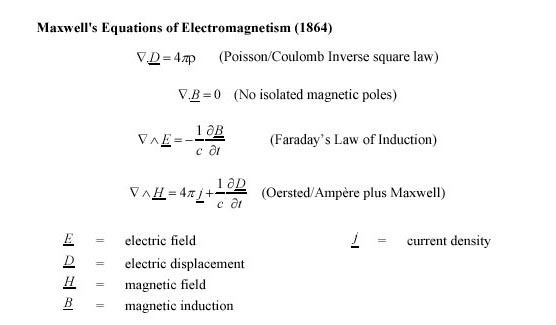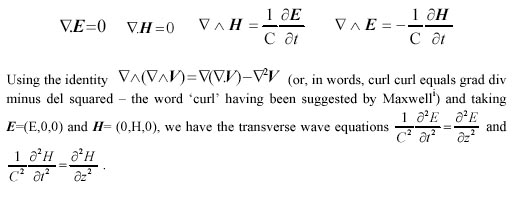It will be seen that Maxwell, as well as "mathematising" the equations of electromagnetism, added only one term to one of the equations but if you have the boldness (or genius) to do so, a wave equation results and the velocity of the wave can be calculated from purely electromagnetic measurements, apparently unconnected with the observed velocity of light.

In a vacuum these equations reduce to:From the extraordinary co-incidence that the velocity of these transverse electromagnetic waves (C, a ratio derived purely from electromagnetic experiments) was, within experimental error, equal to the observed velocity of light, c, Maxwell concluded that:- This velocity is so nearly that of light, that it seems we have strong reason to conclude that light itself (including radiant heat, and other radiations if any) is an electromagnetic disturbance in the form of waves propagated through the electromagnetic field according to electromagnetic laws.

This conclusion of 1865 (i.e. that C=c and light is an electromagnetic wave) was the most stunning triumph of theoretical physics in the 19th century

In 1888, Hertz was able to show electromagnetic waves, of a frequency we would now call radio waves of 6 cm. wavelength, being transmitted across the laboratory (the wavelength of visible light is between 0.44 and 0.77 times 10-6 cms. i.e. very much shorter than that for wireless communication). This was the most stunning triumph of experimental physics in the 19th century.